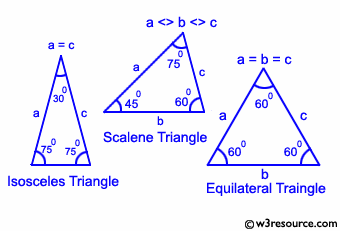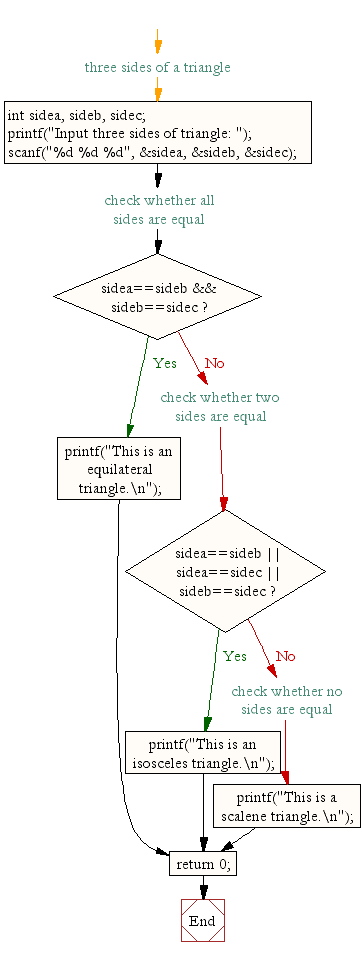﻿ C : Check if a triangle is Equilateral, Isosceles or Scalene# C Exercises: Check whether a triangle is Equilateral, Isosceles or Scalene

## C Conditional Statement: Exercise-14 with Solution

Write a C program to check whether a triangle is Equilateral, Isosceles or Scalene.

Equilateral triangle: An equilateral triangle is a triangle in which all three sides are equal. In the familiar Euclidean geometry, equilateral triangles are also equiangular; that is, all three internal angles are also congruent to each other and are each 60°.

Isosceles triangle: An isosceles triangle is a triangle that has two sides of equal length.

Scalene triangle: A scalene triangle is a triangle that has three unequal sides, such as those illustrated above.

Pictorial Presentation:Sample Solution:

C Code:

``````#include <stdio.h>
int main()
{
int sidea, sideb, sidec; //are three sides of a triangle

/*
* Reads all sides of a triangle
*/
printf("Input three sides of triangle: ");
scanf("%d %d %d", &sidea, &sideb, &sidec);

if(sidea==sideb && sideb==sidec) //check whether all sides are equal
{
printf("This is an equilateral triangle.\n");
}
else if(sidea==sideb || sidea==sidec || sideb==sidec) //check whether two sides are equal
{
printf("This is an isosceles triangle.\n");
}
else //check whether no sides are equal
{
printf("This is a scalene triangle.\n");
}

return 0;
}
```
```

Sample Output:

```Input three sides of triangle: 50 50 60
This is an isosceles triangle.
```

Flowchart:C Programming Code Editor:

Improve this sample solution and post your code through Disqus.

What is the difficulty level of this exercise?

Test your Programming skills with w3resource's quiz.

﻿

## C Programming: Tips of the Day

Why do C and C++ compilers allow array lengths in function signatures when they're never enforced?

It is a quirk of the syntax for passing arrays to functions.

Actually it is not possible to pass an array in C. If you write syntax that looks like it should pass the array, what actually happens is that a pointer to the first element of the array is passed instead.

Since the pointer does not include any length information, the contents of your [] in the function formal parameter list are actually ignored.

Ref : https://bit.ly/3fhlvdH